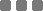## Friday, November 15, 2013

### Math: Basic Trigonometric Functions and Special Angles and moar..

In mathematics, the trigonometric functions (also called the circular functions) are functions of an angle. They relate the angles of a triangle to the lengths of its sides. Trigonometric functions are important in the study of triangles and modeling periodic phenomena, among many other applications.
Dear Wikipedia

Basic : Sin, Cos, Tan
Let's take a look at a right angled triangle (a triangle that has a right angle : 90°) below :
And let's take the triangle's corner A as our angle reference.

SINE function (abbreviated as sin) is the length ratio between the opposite (a) and the hypotenuse (h) side.
Sin A = opposite (a) / hypotenuse (h)

COSINE function (abbreviated as cos) is the length ratio between the adjacent (a) side and the hypotenuse (h).
Cos A = adjacent (b) / hypotenuse (h)

TANGENT function (abbreviated as tan) is the length ratio between the opposite (a) side and the adjacent (h) side.
Tan A = opposite (a) / adjacent (b)

It also can be written as the ratio of sin and cos functions :
Tan A = Sin A / Cos A

Reciprocal functions : Cosec, Sec, Cotan
COSECANT function (abbreviated as csc or cosec) is the length ratio between the hypotenuse (h) and opposite (a) side.
Cosec A = 1 / Sin A = hypotenuse (h) / opposite (a)

SECANT function (abbreviated as sec) is the length ratio between the hypotenuse (h) and the adjacent (a) side.
Sec A = 1 / Cos A = hypotenuse (h) / adjacent (b)

COTANGENT function (abbreviated as cotan or cot) is the length ratio between the adjacent (h) side and the opposite (a) side.
Cot A = 1 / Tan A = adjacent (b) / opposite (a)

It also can be written as the inverted ratio of sin and cos functions :
Cot A = Cos A / Sin A

Inverse function : the degree/rad value
To find the angle value on each, we can use the inverse functions of the trigonometric functions above (with restricted domains). The inverse functions of those are noted with arc or the exponent -1 on the function.
Such as : arcsin (value) or sin-1 (value),
which resulting the corresponding angle of the function value :
arcsin (sin value) = deg/rad value

For example, arcsin (½) or sin-1 (½) = 30° = π/6 rad

Special angles values
This is the table of special angles in Trigonometry:
 func. deg 0° 30° 45° 60° 90° 180° Sin 0 ½ ½√2 ½√3 1 0 Cos 1 ½√3 ½√2 ½ 0 -1 Tan 0 ⅓√3 1 √3 ~ 0 Csc ~ 2 √2 ⅔√3 1 ~ Sec 1 ⅔√3 √2 2 ~ -1 Cot ~ √3 1 ⅓√3 0 ~

For instance :
• 60° = 60°/180° * π = (1/3)π rad
• (1/2)π rad = [ (1/2)π * 180° ]/π = 90°

This is a fun example of using Trigonometry and Pythagorean for finding direction (angle) and distance from the point origin, respectively.

It's using CSS for styling the elements and jQuery (JavaScript) to do the basic functions.

Start by clicking this window below (please use Chrome or Firefox browser) :

See the Pen Laser Compass by Monkey Raptor (@monkeyraptor) on CodePen

• The x and y coordinates of the clicking point are captured using jQuery `pageX` and `pageY` events. Of course, with a bit of manipulation, because the center of the coordinate isn't on top left of the screen (window).
• I measure the distance (hypotenuse) using Pythagorean, that is : distance = √(x² + y²).
• For the angle (direction), I use arccos of the (x coordinate/distance) value. It's using JavaScript `Math.acos()`. Then round the result using `.toFixed()`. That's maybe the main cause of the not-so-precise result, the rounded digit.
• The angle (deg) result is then targeted to the dude (center) element, specifically on the CSS3 `transform:rotate(value)` property.
• And the last but not least, I also put some CSS transition effects (triggered via jQuery) to make it more dramatic. ^_^
• I use PrefixFree.js (which can be omitted by the way, probably) and Normalize.css on that demo.
You can examine how I coded them there (on the JS tab) and see the CSS styling. You may use and improvise this to make it more advanced and precised.Math: Basic Trigonometric Functions and Special Angles and moar..
https://monkeyraptor.johanpaul.net/2013/11/basic-trigonometric-functions-and.html